# Speech controlled math test with Advanced Composer

## Contents

• Use a loop block

• Use a random number block

• Use a math formula block

• Use a wait for voice command block

• Use an if/else block

For this tutorial we’ll assume that you already know how to navigate to the Advanced Composer inside the ZBOS Control application. This tutorial starts in the Advanced Composer with a new and empty composition.

### Scenario

We’ll make a composition to start a math quiz. This quiz will ask the user 10 random math questions where it will ask to add up two numbers. After each question the robot will provide the user with some feedback if the result was correct or not.

## Step-by-step guide

### Start block

1. Search the Start block in the menu on the left and click on it or drag it to the work field. A start block is obligatory for every advanced composition, this is always the first block of each advanced composition.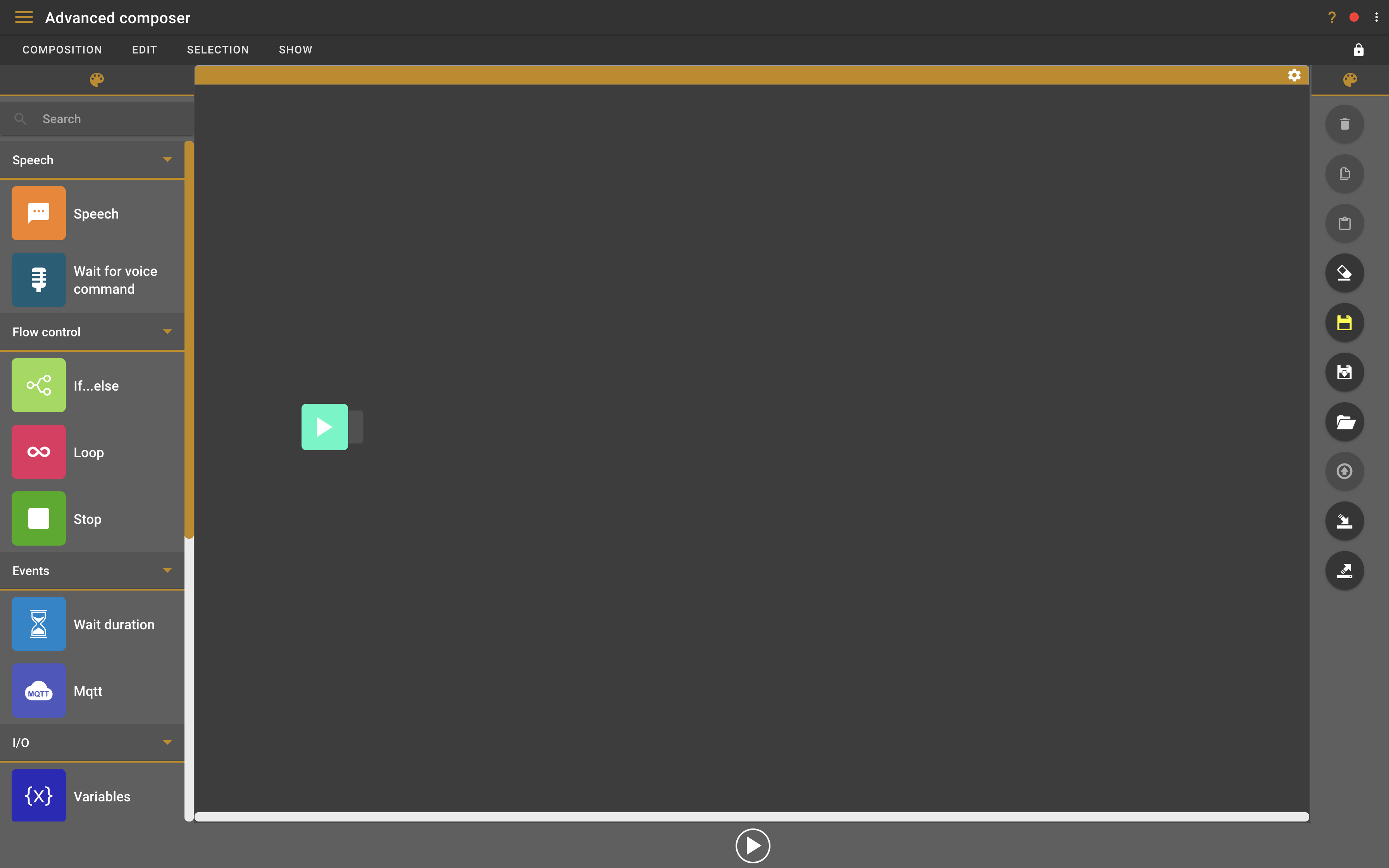### Loop block

1. Search the Loop block in the menu on the left and click on it or drag it to the work field. The loop block will tell the composition to repeat a certain action or part of the composition for a certain amount of times.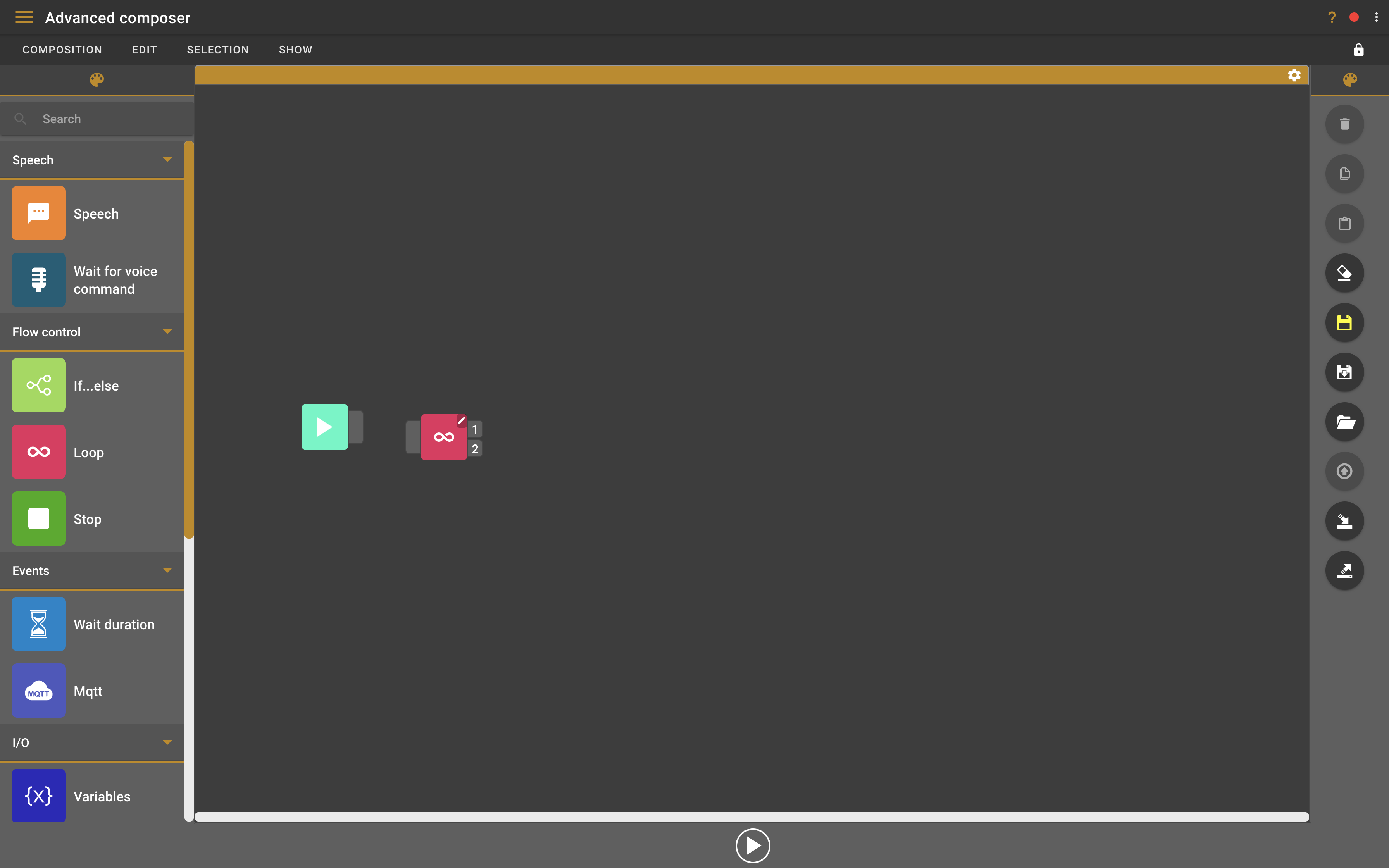2. Double-click on the loop block to configure it. This will open a small settings screen.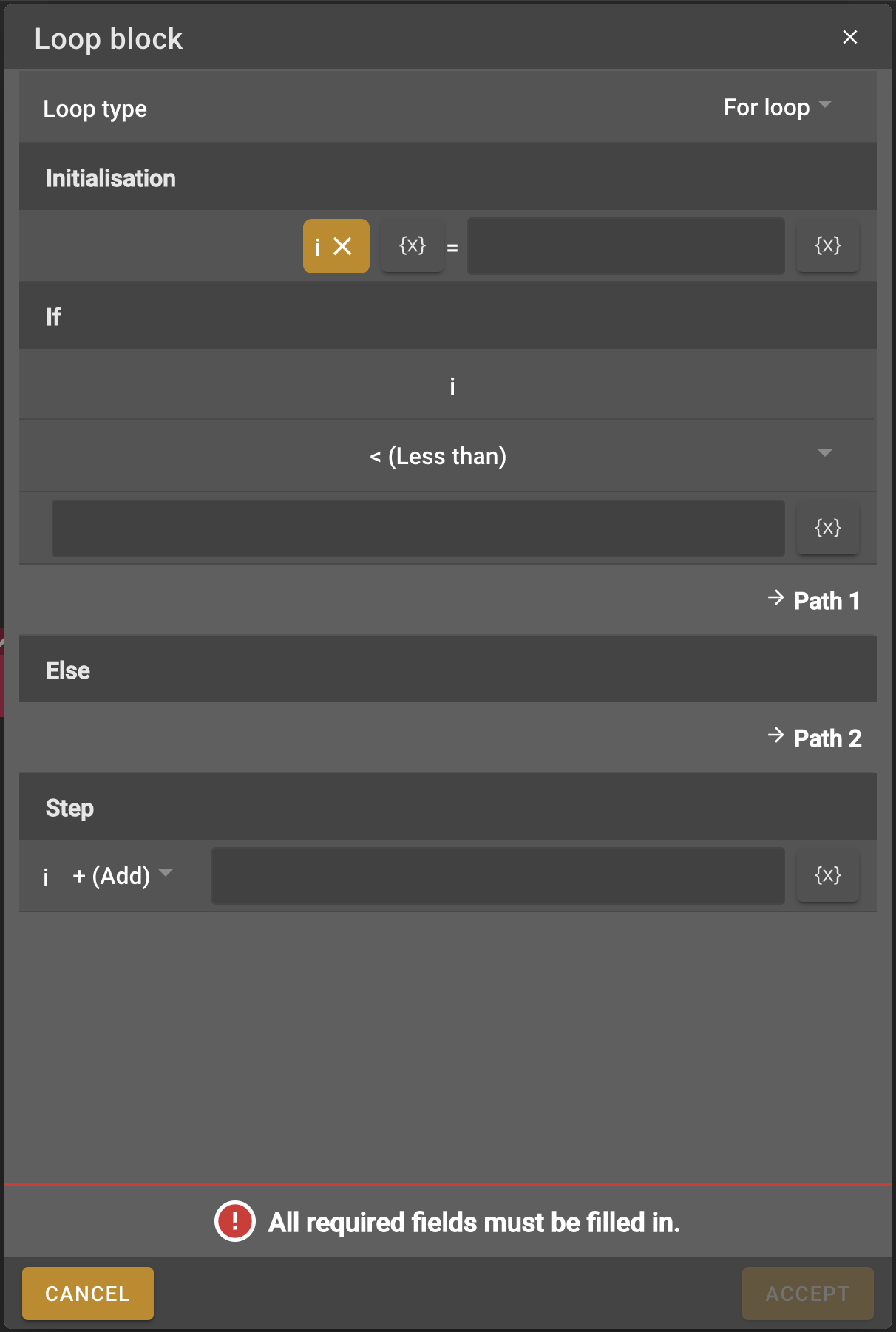3. There are two main loop options for the loop block, which will run repeatedly until a certain condition has been satisfied. The first option is to use a for loop, which will increment a counter by one (for example). The second option is a while loop, which will continue until a condition has been met. For our composition we will use a for loop and run the rest of the blocks in the composition 10 times.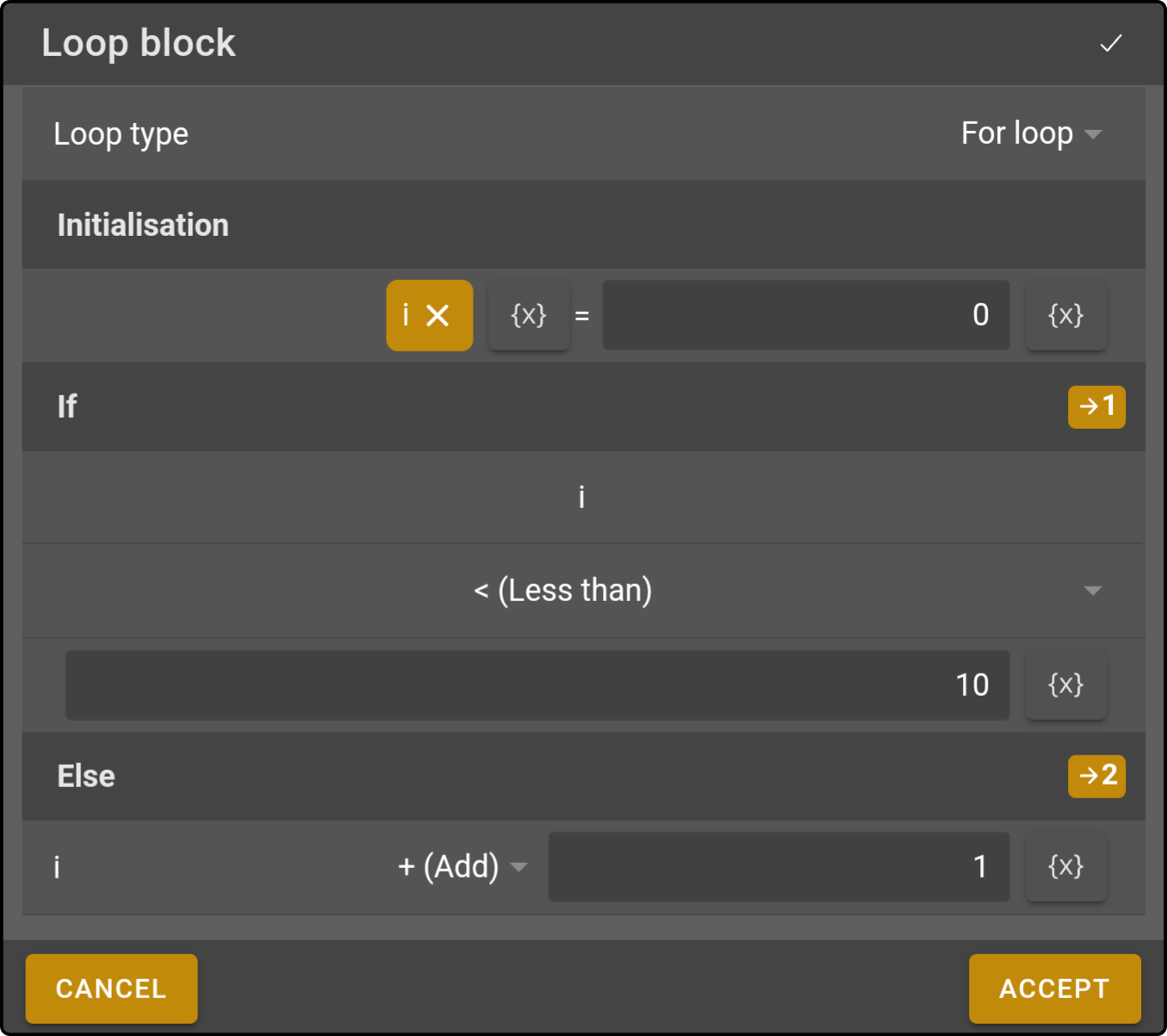1. To make the loop block the second block in the composition we need to connect it to the start block. You can do this by first clicking the node on the right side of the start block and then clicking the node on the left side of the loop block. You will see that a connection appears between these two blocks.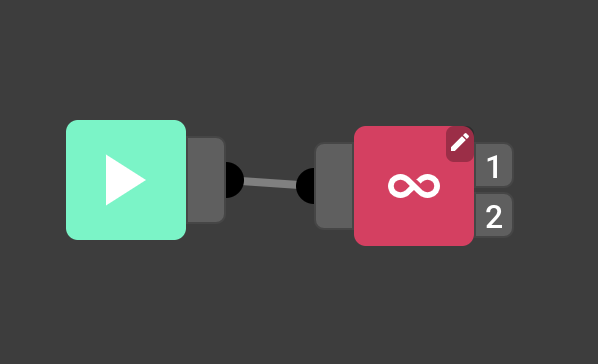2. The loop block has two nodes on the right side. The first node is the part of the composition that you want to loop through. The second node is the part of the composition that will start when you exit the loop. We will first build onto the first node, the one that will be looped.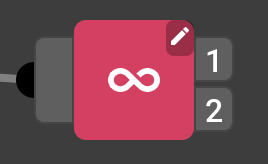### Random number block

1. We want the robot to ask different numbers each time, to do this we will use a Random number block. Find this block in the left menu and drag it onto the work field.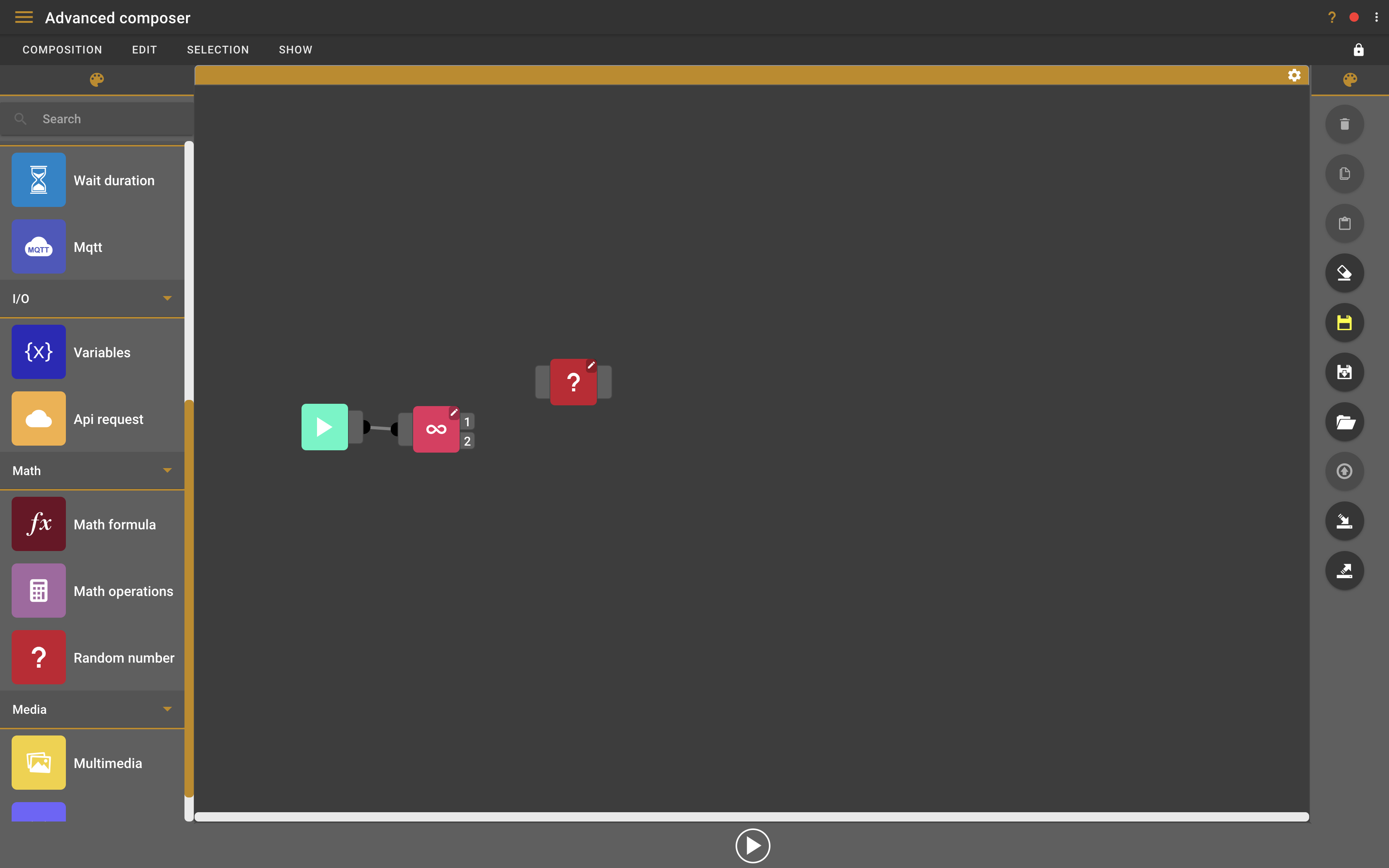2. Double-click on the loop block to configure it. This will open a small settings screen.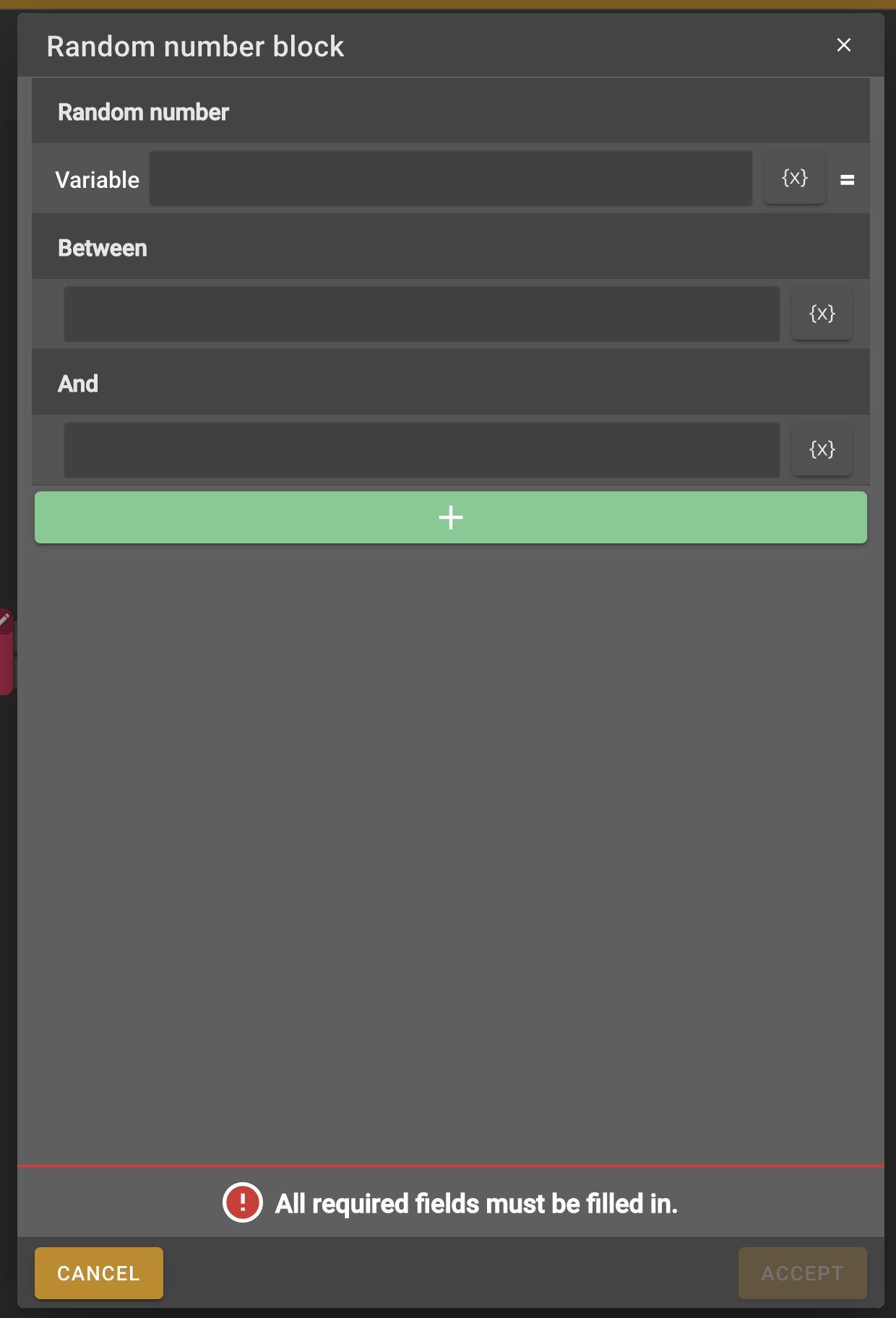3. We need to give the random number block a variable name, this way we can use the value in upcoming blocks. We also need to provide the minimum and maximum value that the random number can be. In our case we call the variable "number1", and the random number will have to be between 0 and 10.

4. To do simple math question we will need a second variable. To do this we can use the same random number block, in the settings we just click the add button and we can add another variable. We’ll call this variable "number2" and give it the same range of 0 and 10.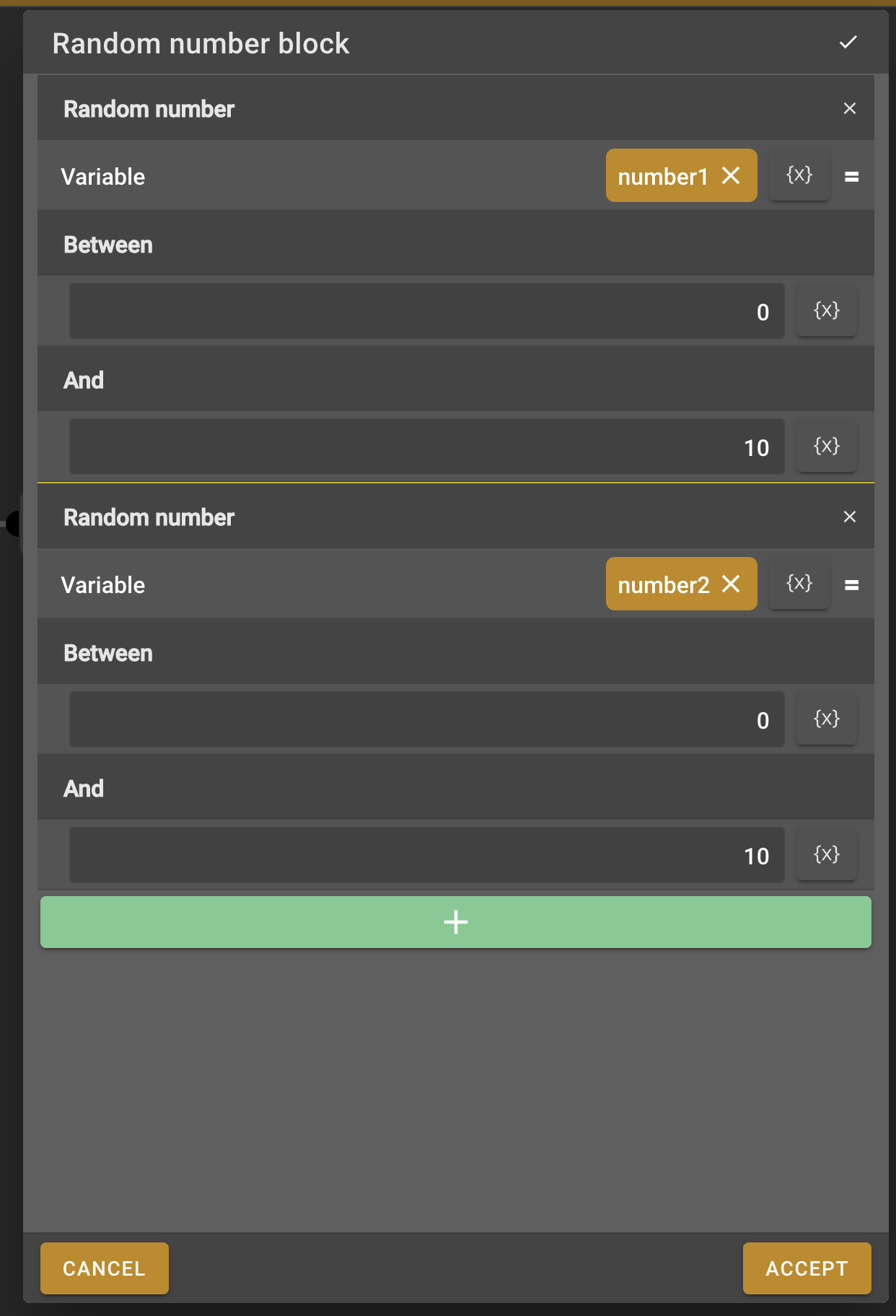5. Now connect the first node of the loop block to the random number block.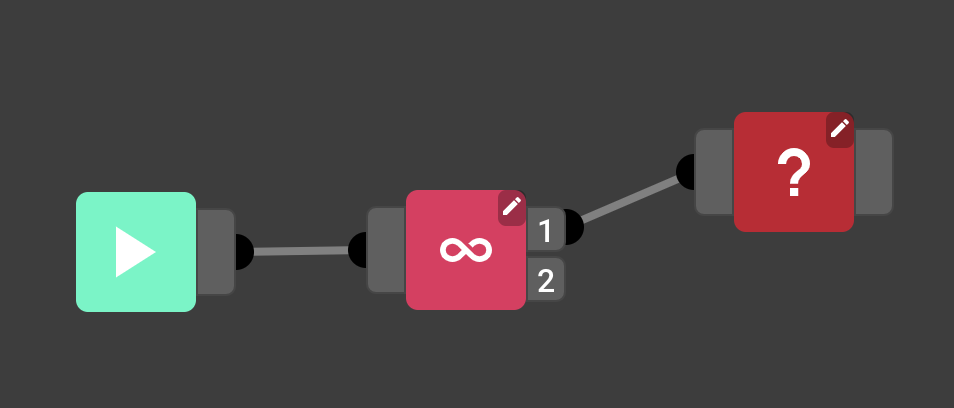### Speech block with variables

1. Now we want the robot to ask the user the math question using the previously defined random numbers. For this we will need a Speech block, find it in the menu on the left and add it to the work field.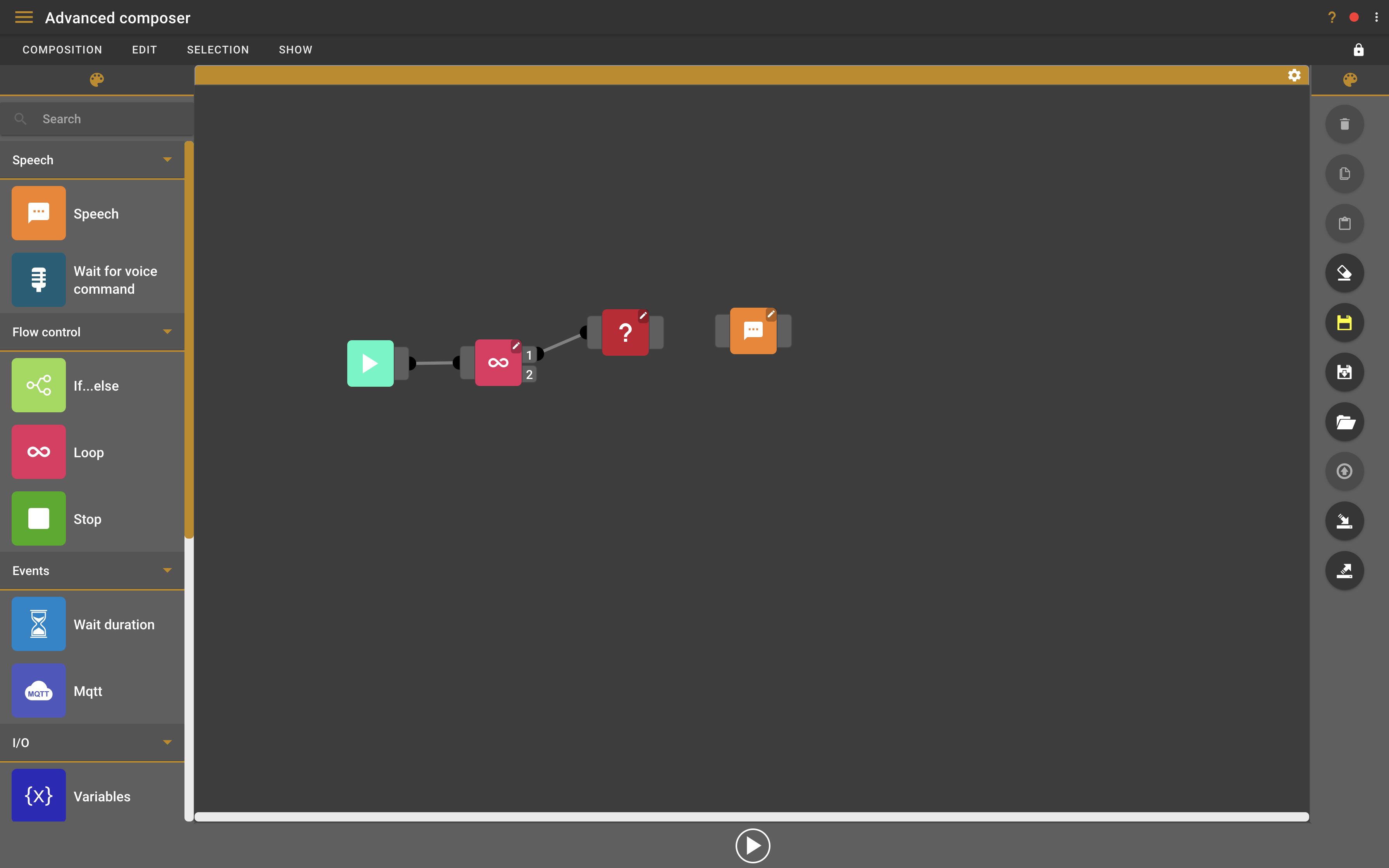2. To be able to access the variables from the random number block we will need to connect the random number block to the speech block. Connect these two blocks like you did for the previous ones.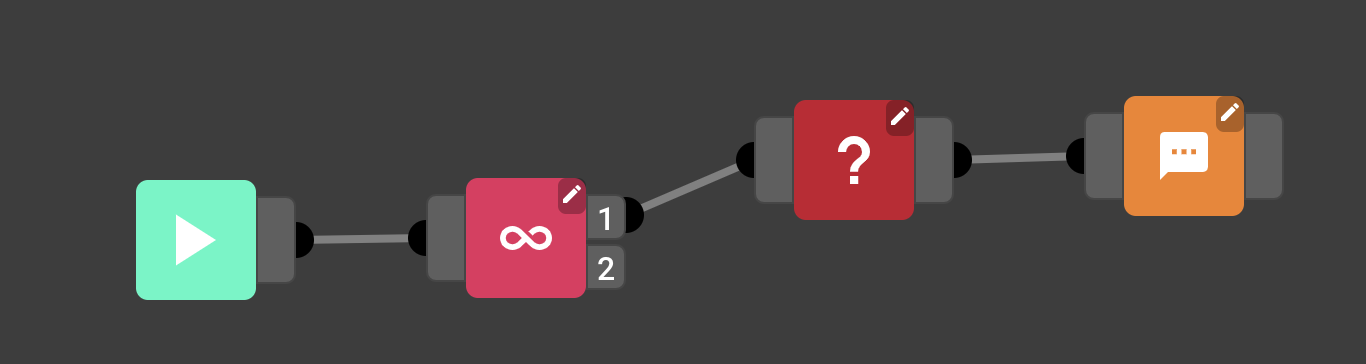3. Now that the speech block is connected to the random number block double-click the speech block to open the speech block settings.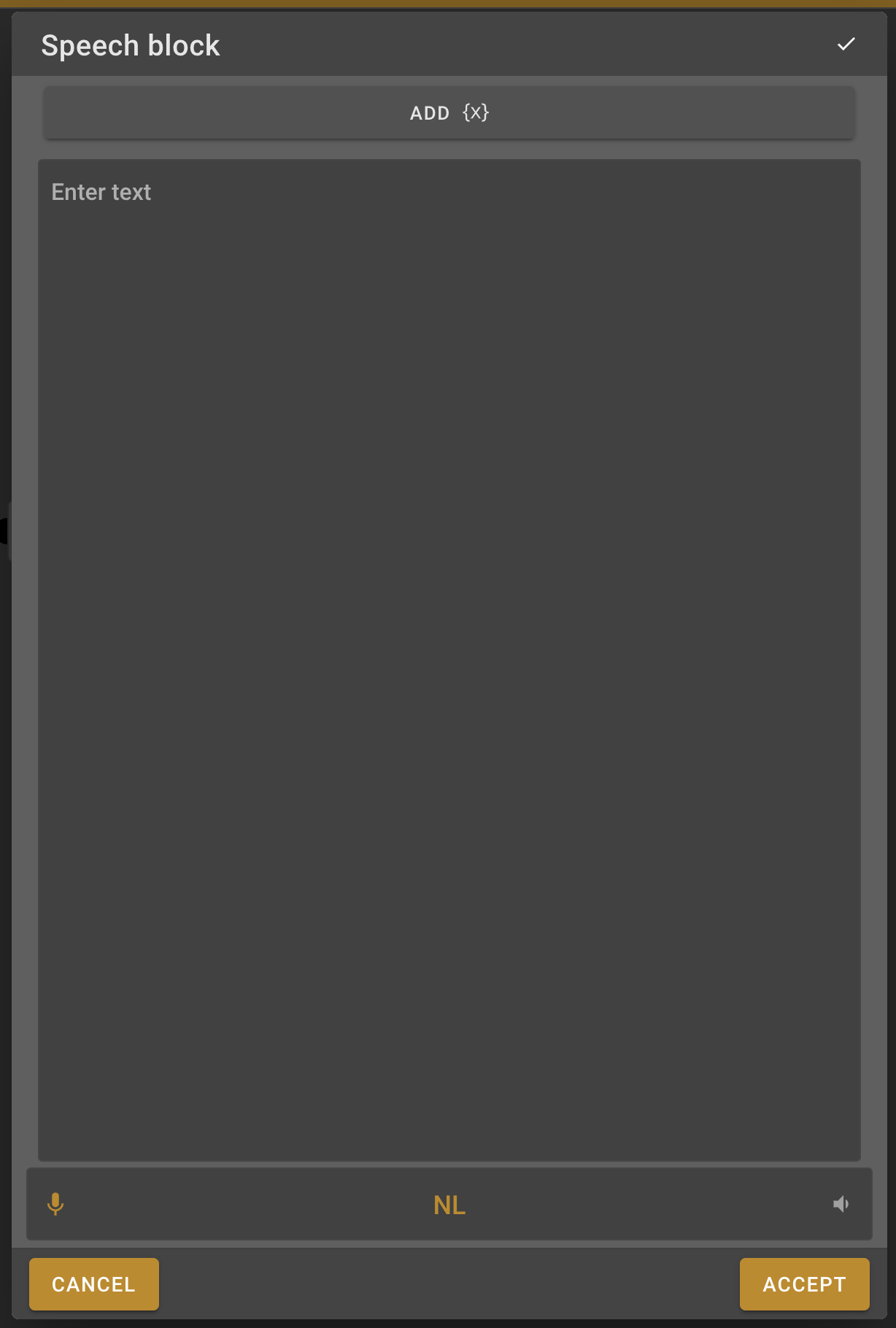4. Use the "Add {x}" Button to add the variables to the message field or type {number1} and {number2} in the message box to add them directly.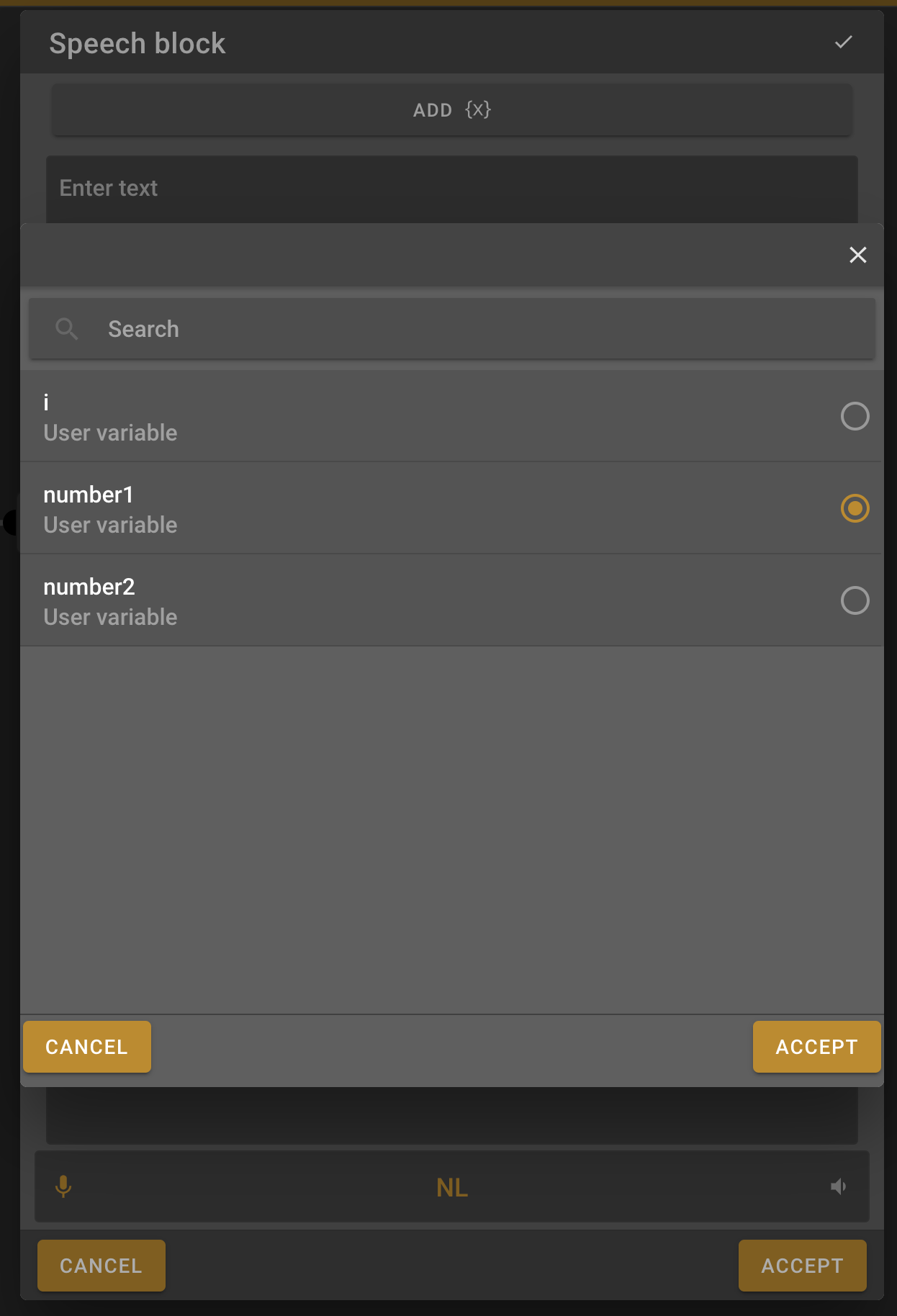5. Type the message that you want the robot to say. In this case I’ll use "How much is {number1} plus {number2}" where {number1} and {number2} are the random numbers from the previous block.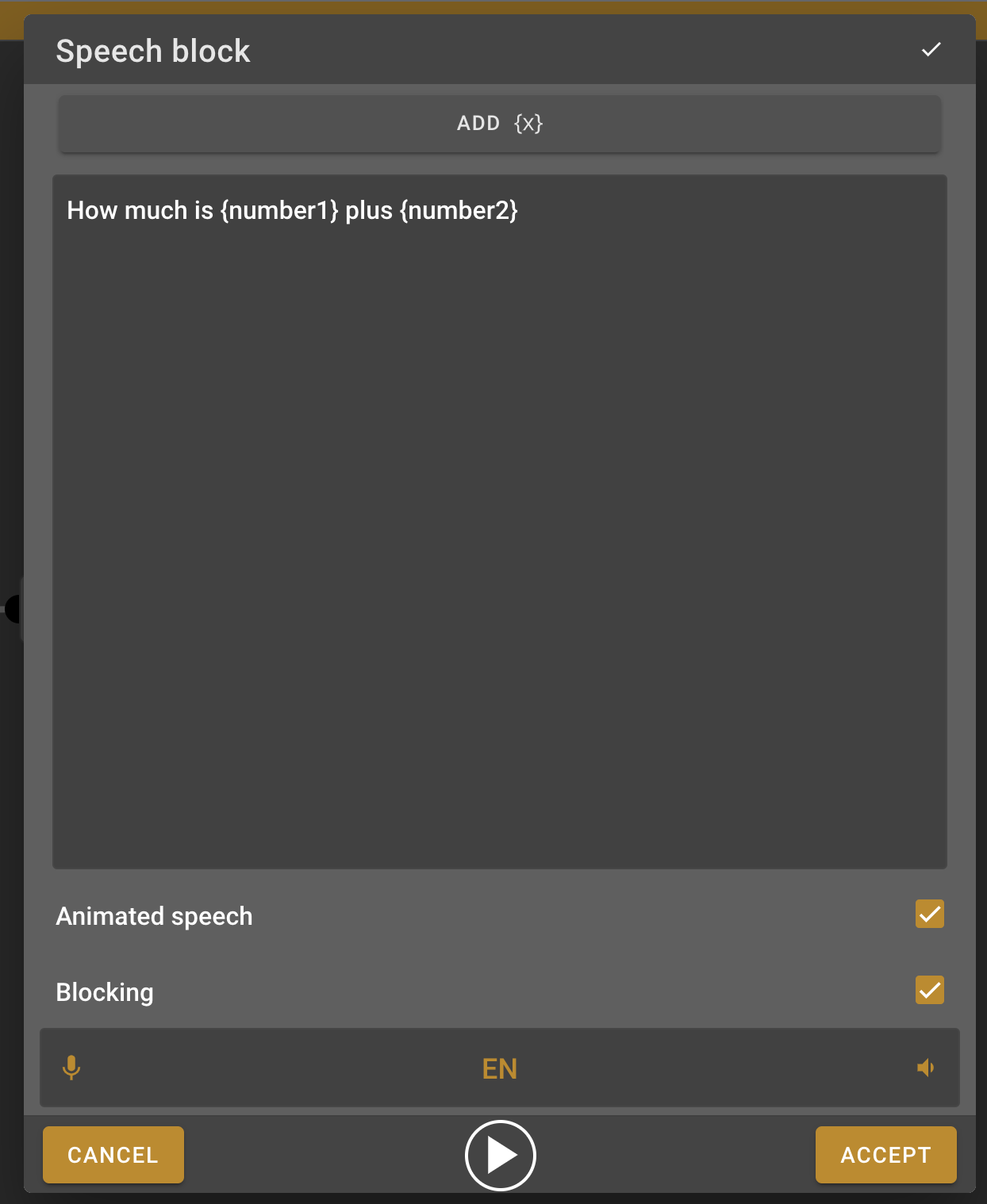### Math formula block

1. To know if the answer that the person will give is correct we need the robot to add the two numbers and save the result in another variable. For this we will need the Math formula block, find this block in the menu on the left and at it to the work field.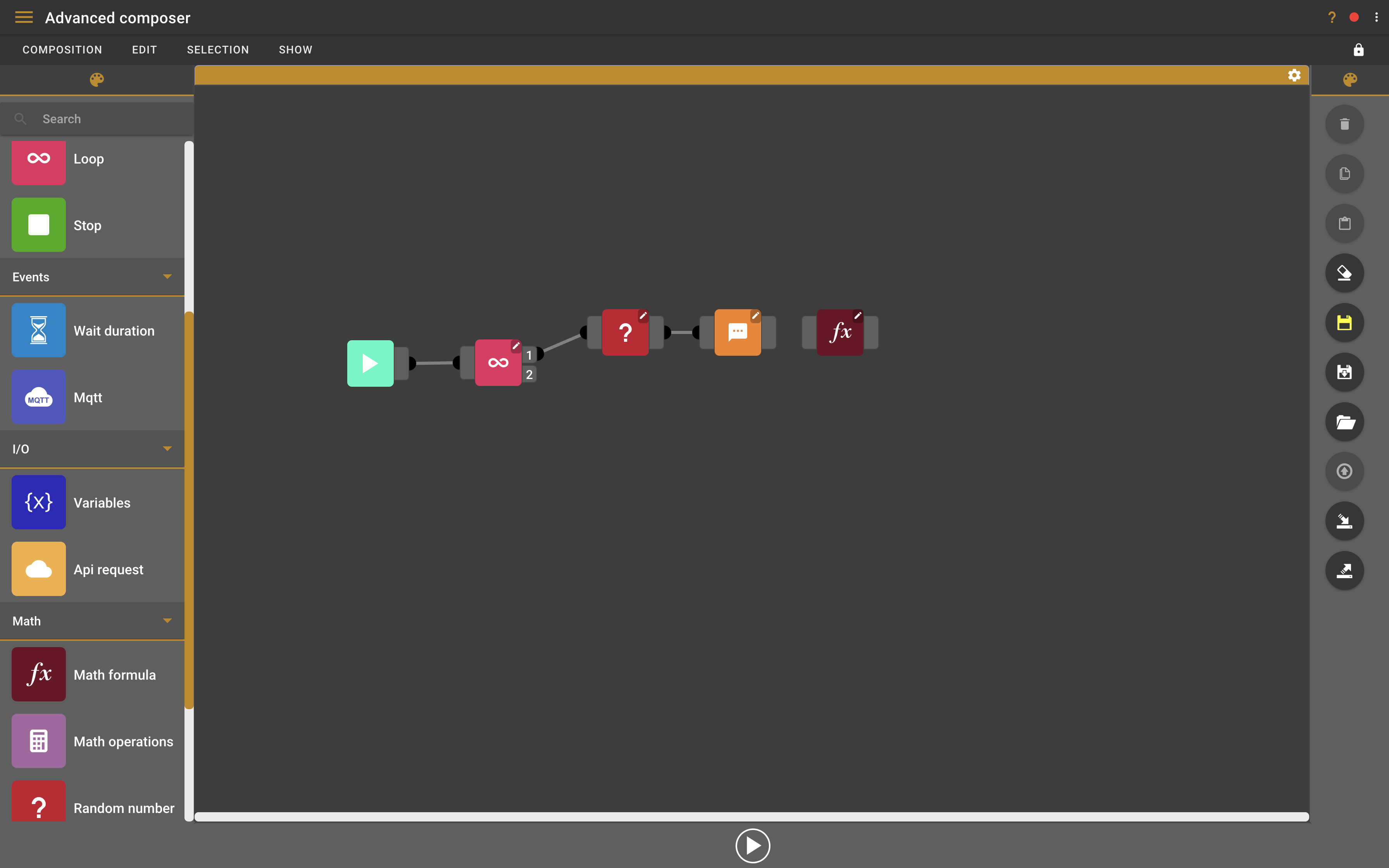2. Connect the Math formula block to the speech block, this way it will be able to access the random number variables from the random number block.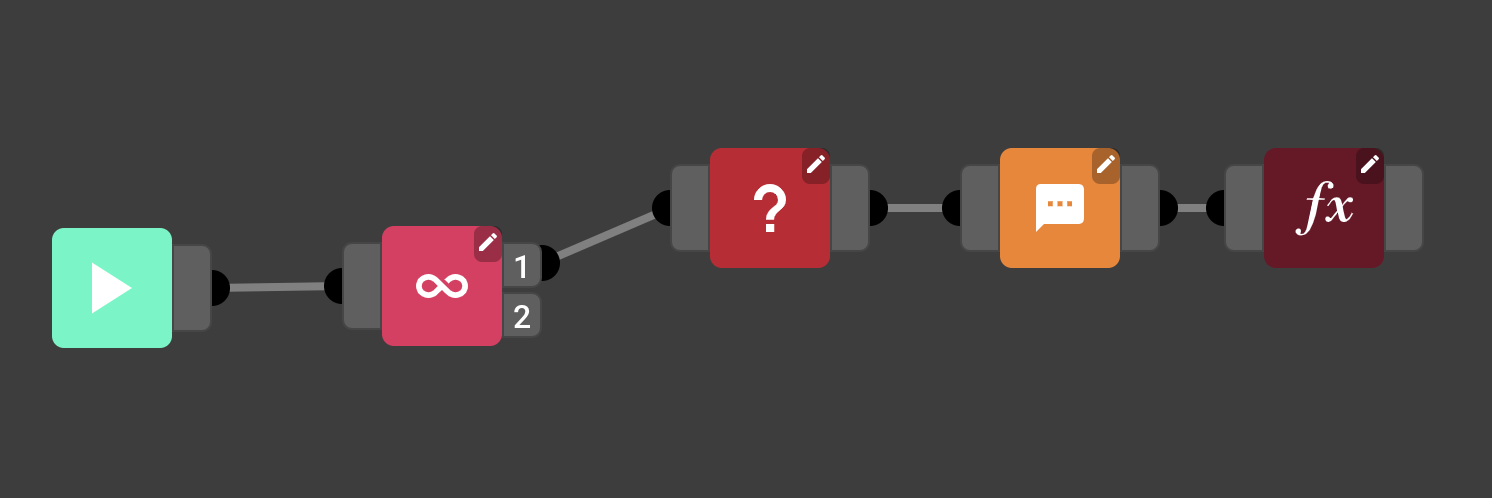3. Now we need to let the Math formula block know which formula it wants to use. Double-click the math formula block to open the settings.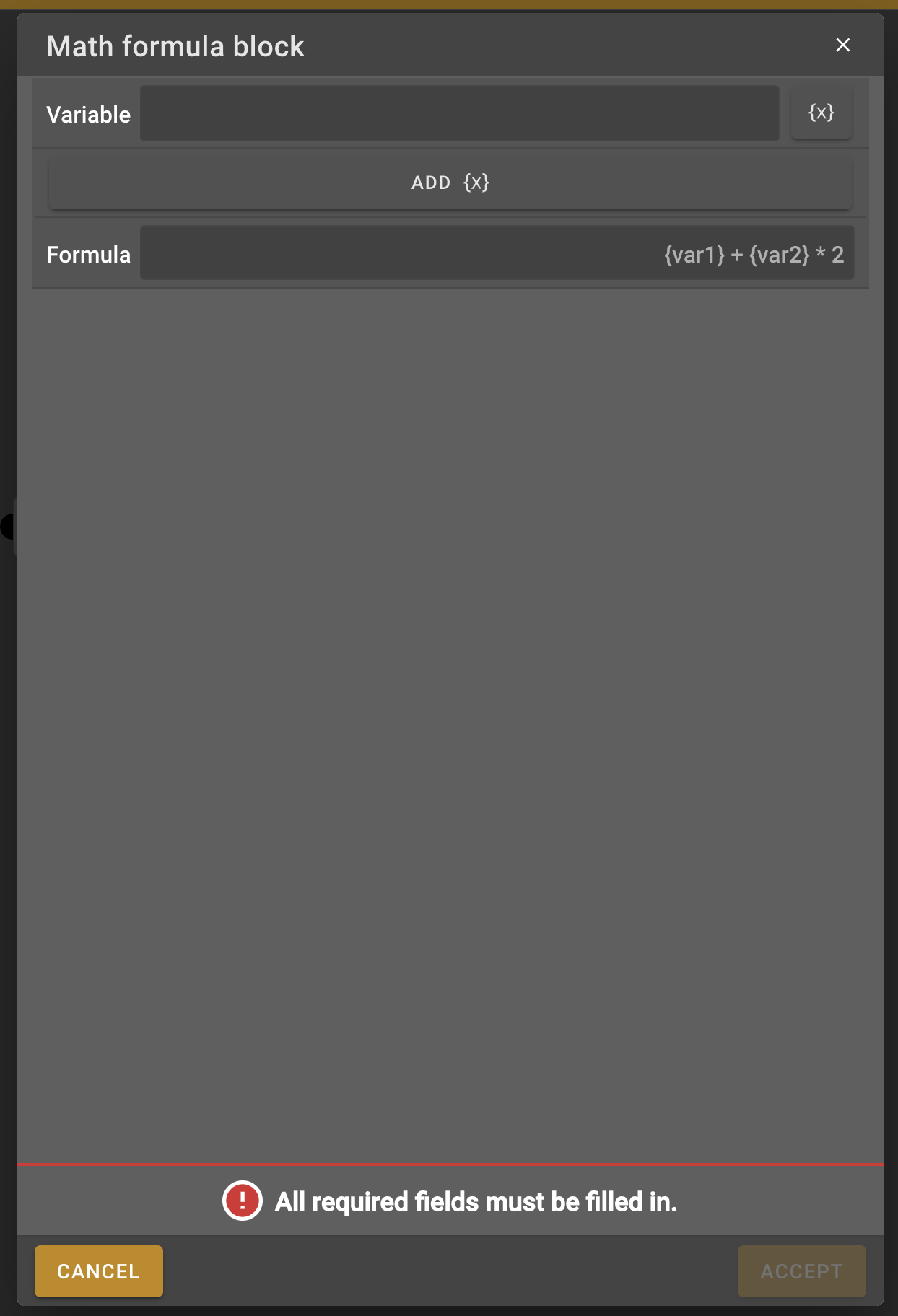4. In the variable text field you can choose a name for the result. In this case because this is the result for the formula I will simply call it "result" but you can choose any name. Now write the formula to add the two random numbers. You can enter the variables the same way as you did for the speech block, write the formula like this "{number1} + {number2}".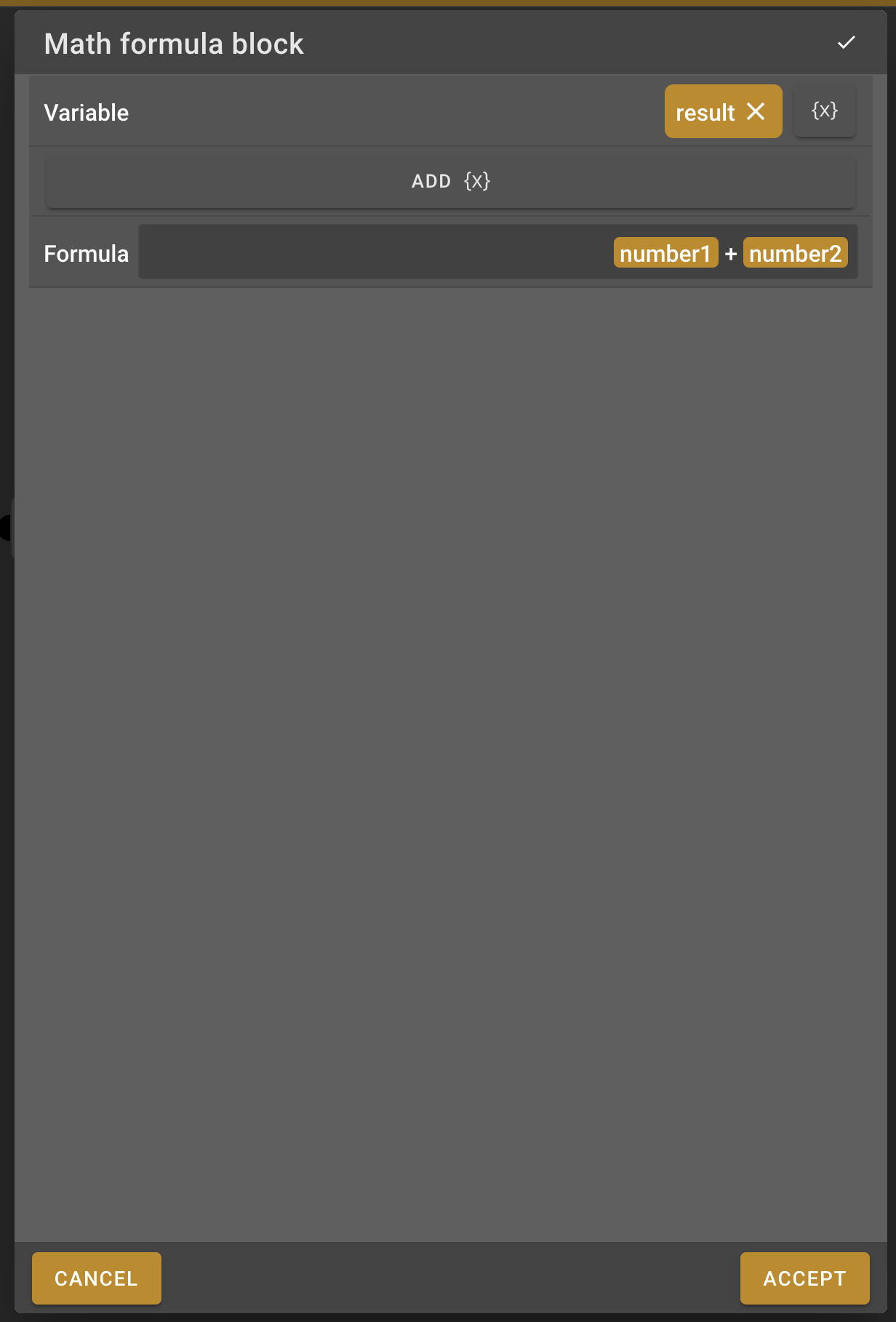### Wait for voice command block

1. Now we need to listen to the user input to check their answer. This can be done using a Wait for voice command block, find this block in the left side menu and put it on the work field.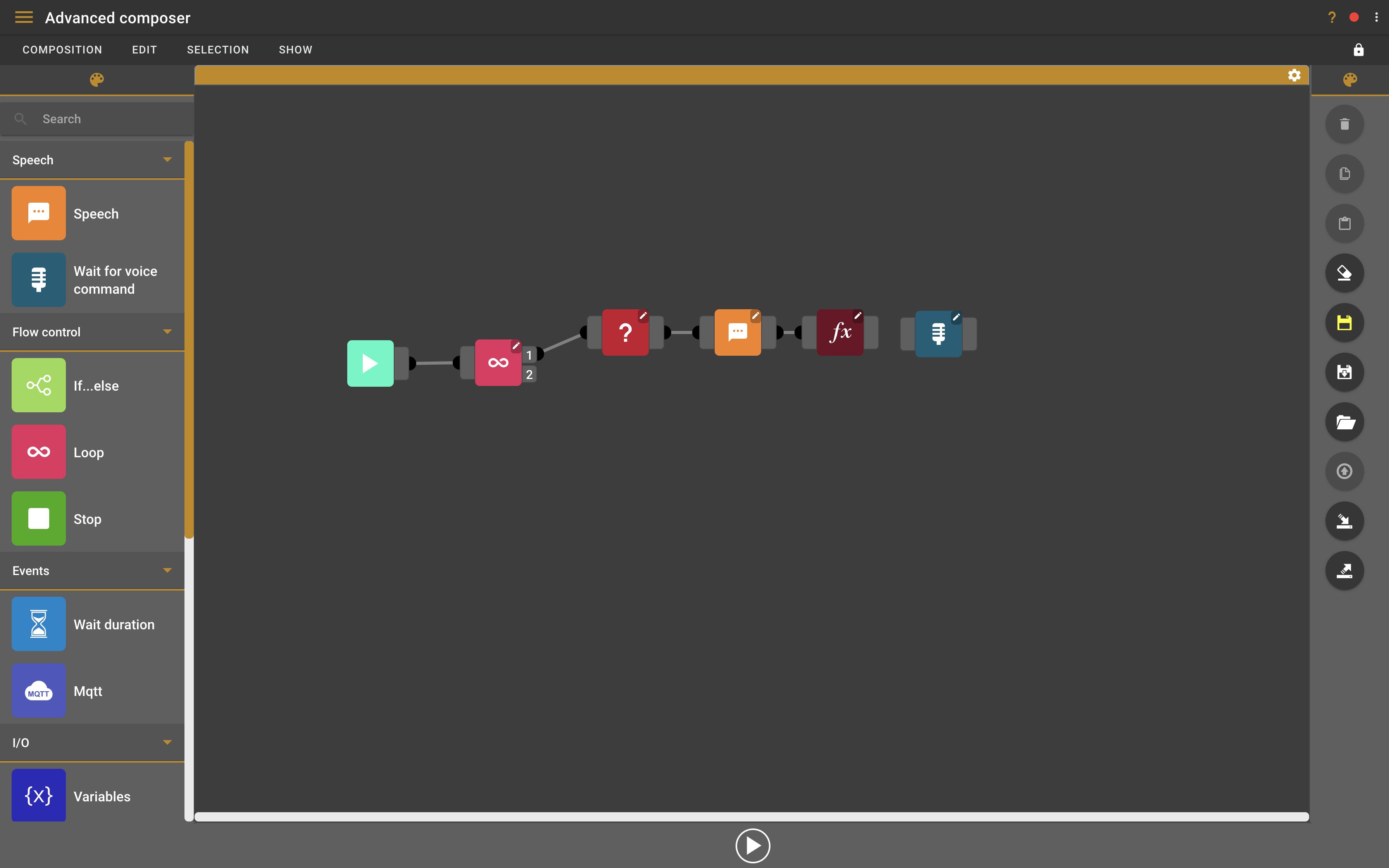2. Connect the wait for voice command block to the math formula block.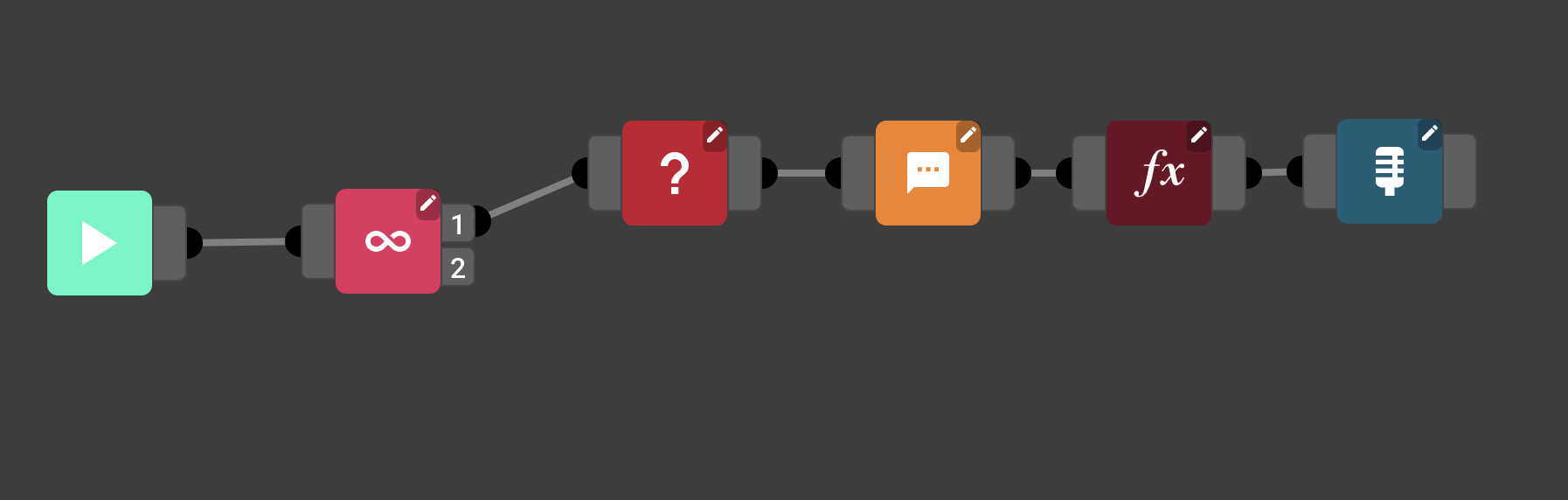3. Double-click the wait for voice command block to open the settings.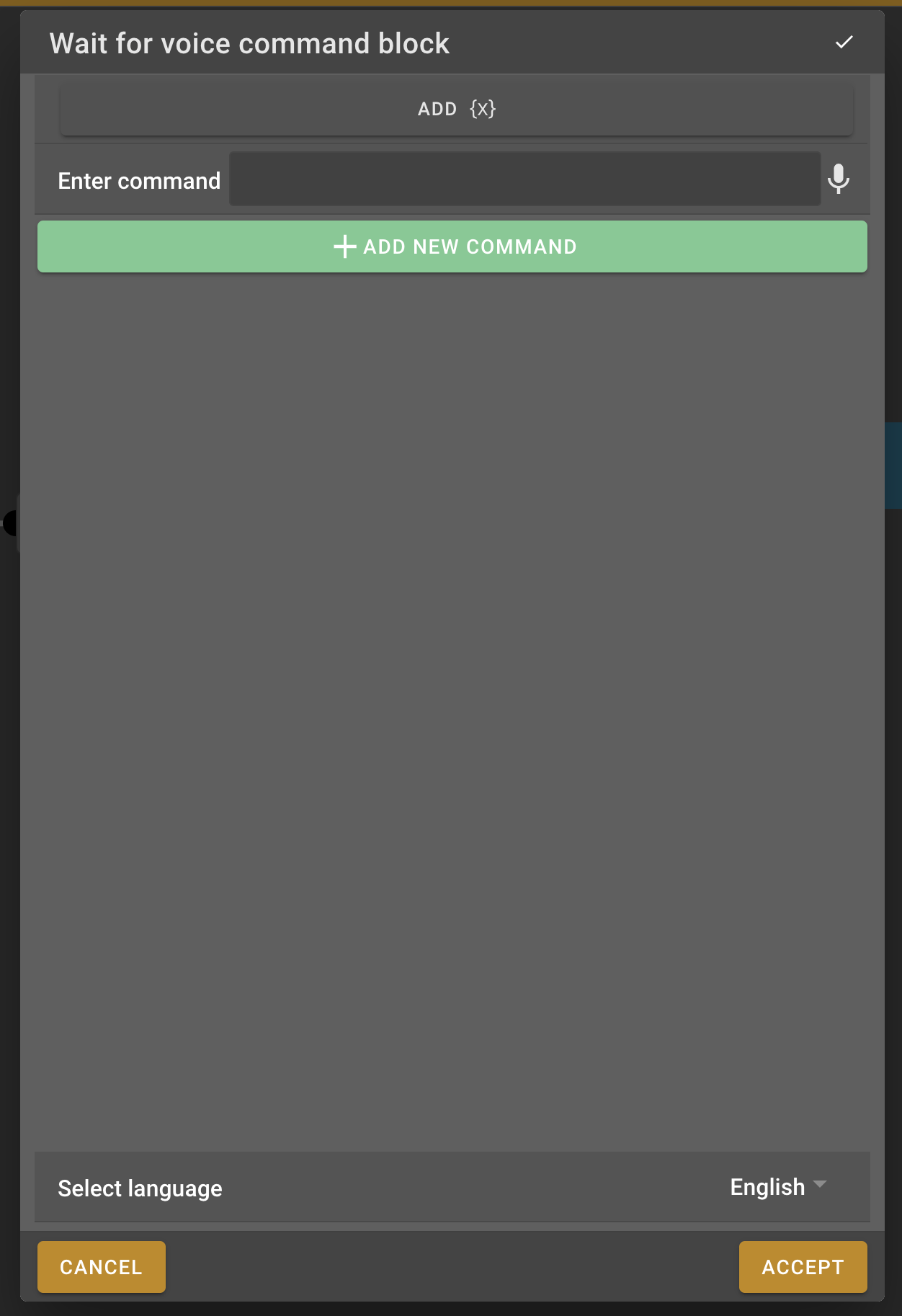4. Now we need to add all the possible correct answers to the wait for voice command settings, this way the robot will know what to listen for. In our case we will have to add the numbers 0 to 20 because those are all the possible correct results for adding two random numbers from 0 to 10.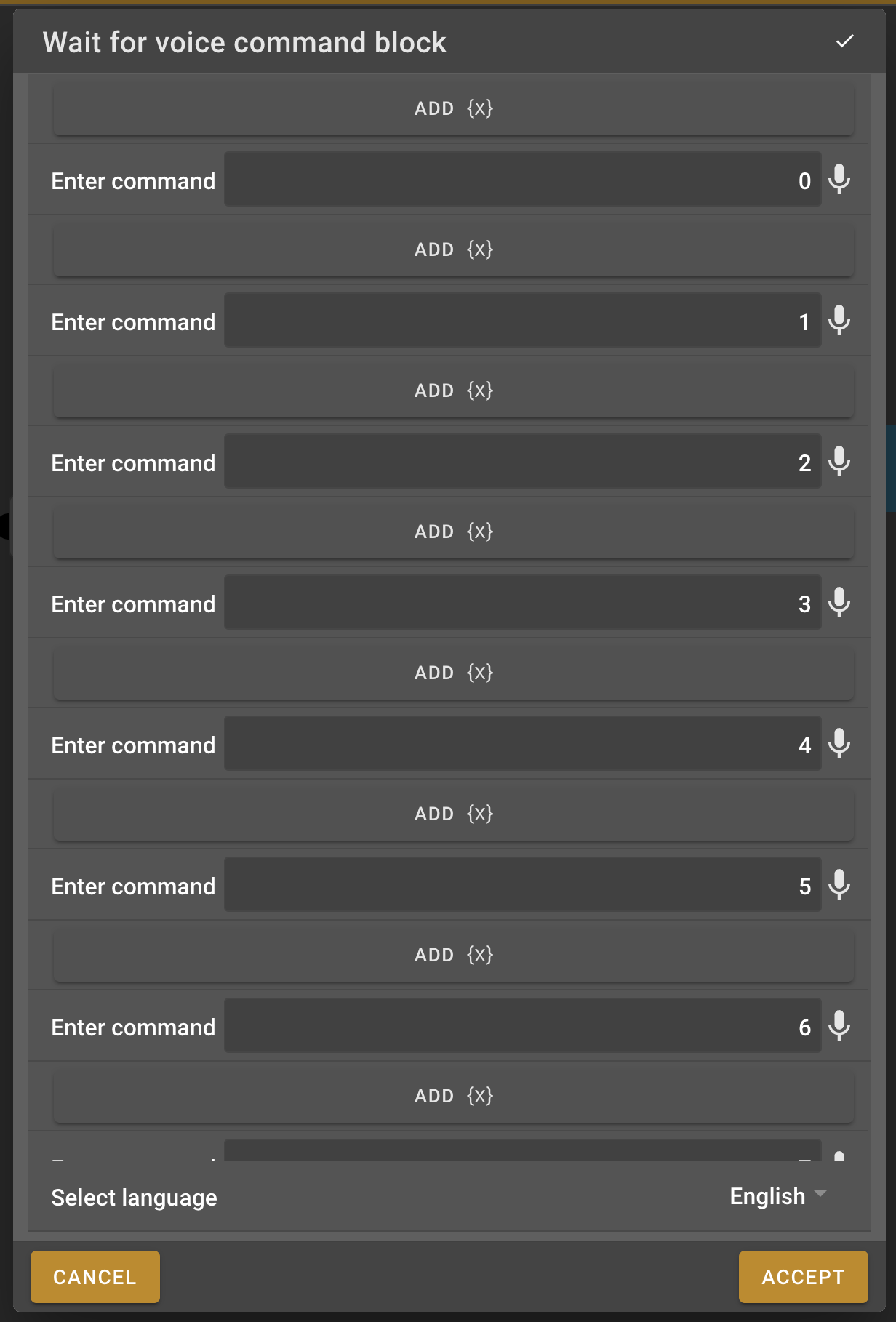### If…​else block

1. Once we heard a valid voice command we will have to determine if it was correct or not. For this we will use the if…​else block. Search it in the menu on the left and put it on the work field.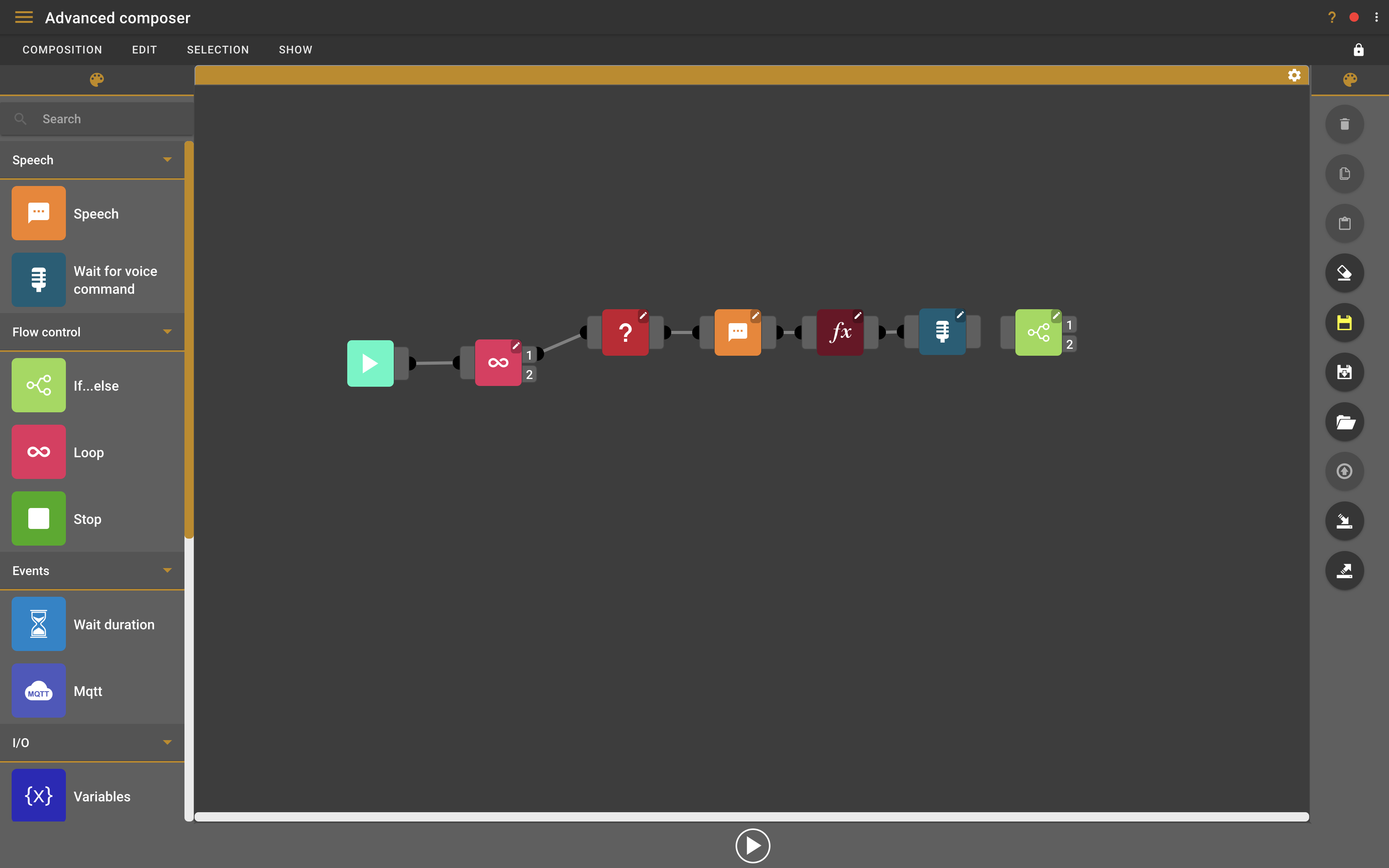2. Connect the if…​else block to the wait for voice command block.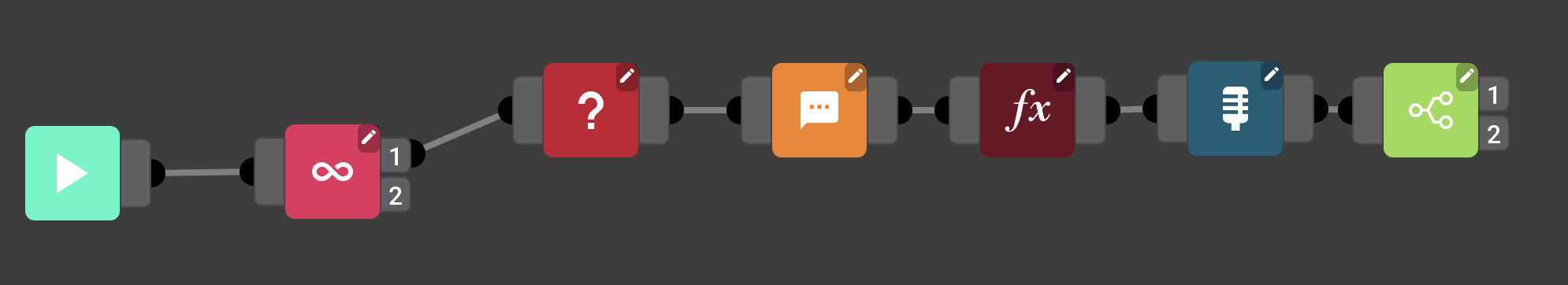3. The if…​else block will check if a certain condition is true. If it is true it will take one path, if not it will take the other. You can see the if…​else block has two nodes on the right side of the block. If the condition is true it will take node 1, if not it will take node 2.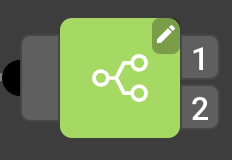4. Double-click the if…​else block to open the settings.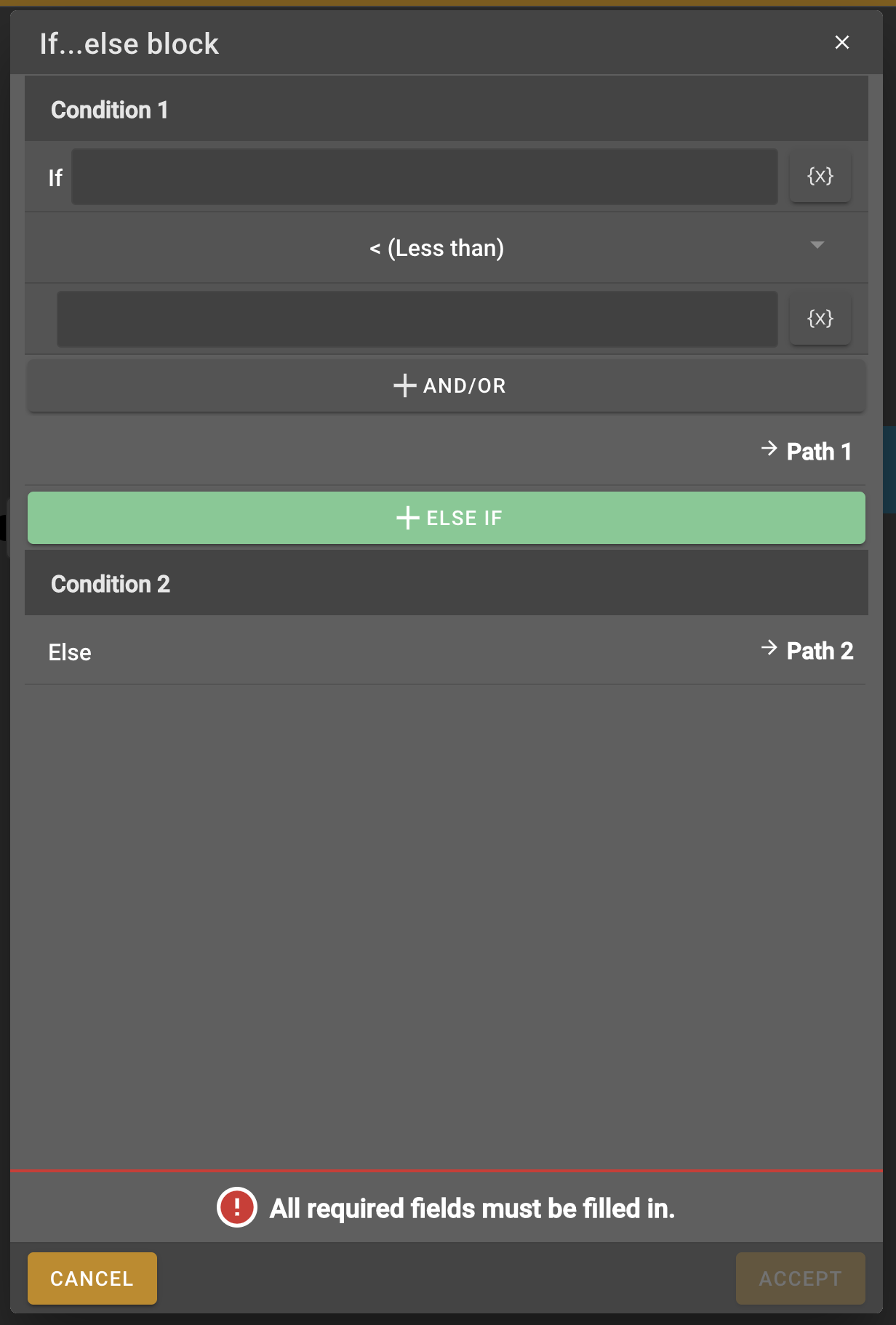5. We want to check if the voice command is the same as the math formula result. We’ll first change the condition to "== (equal to)" because we want to check if the result equals the voice command of the user. Now we will fill in the variables for the voice command and the result in the first and second text field. You can do this the same way as we did with variables before. To use the voice command as a variable you can just select "voice" from the variable list or type {voice} in the text field.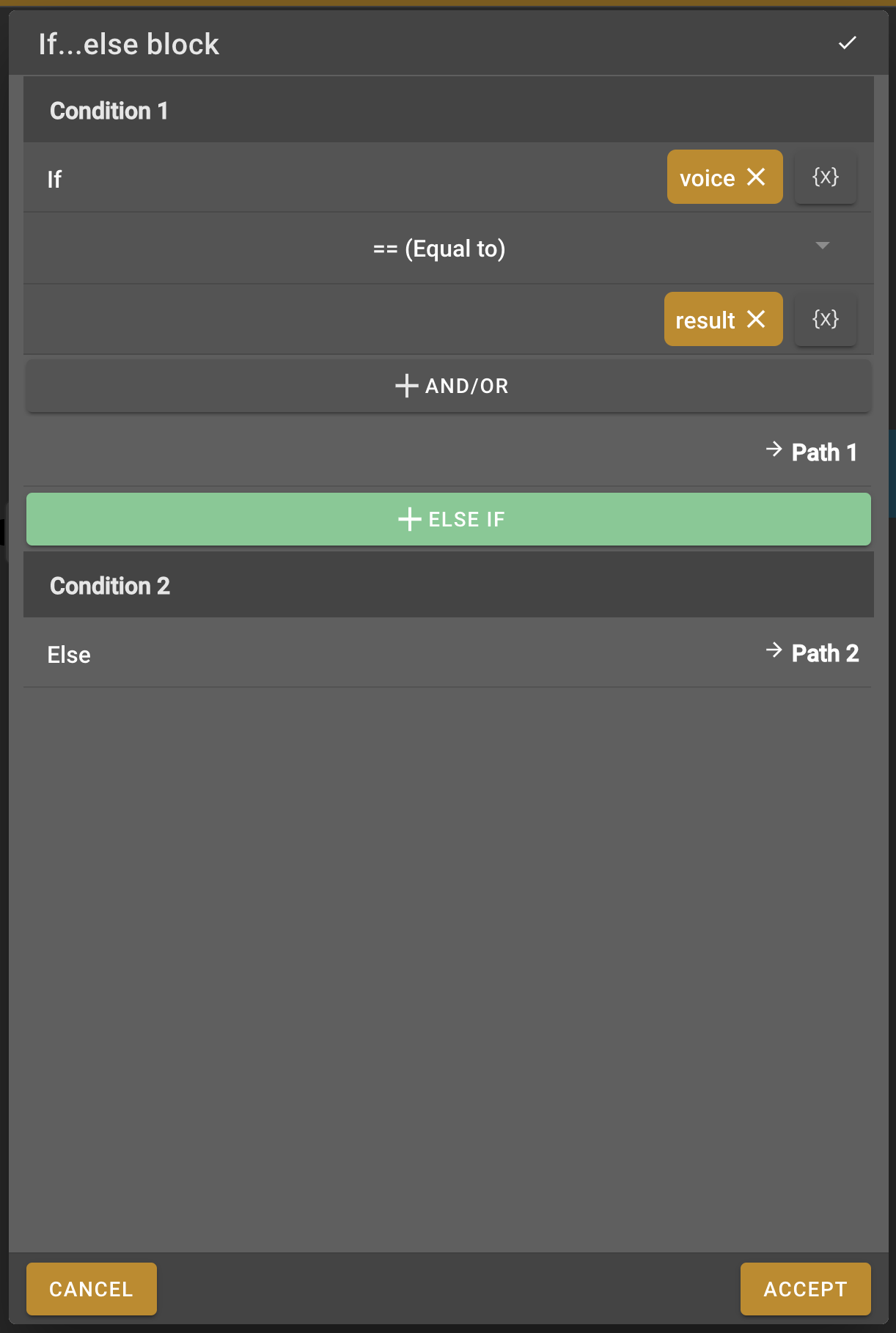6. We want to let the user know if the result was correct or not. If the condition in the if…​else block is true it means that the result was correct. This means that the path continuing from node 1 will be for a correct result and node 2 will be for an incorrect result. We will add two speech blocks onto the work field.

7. We will connect one speech block to the first node and the other one to the second node.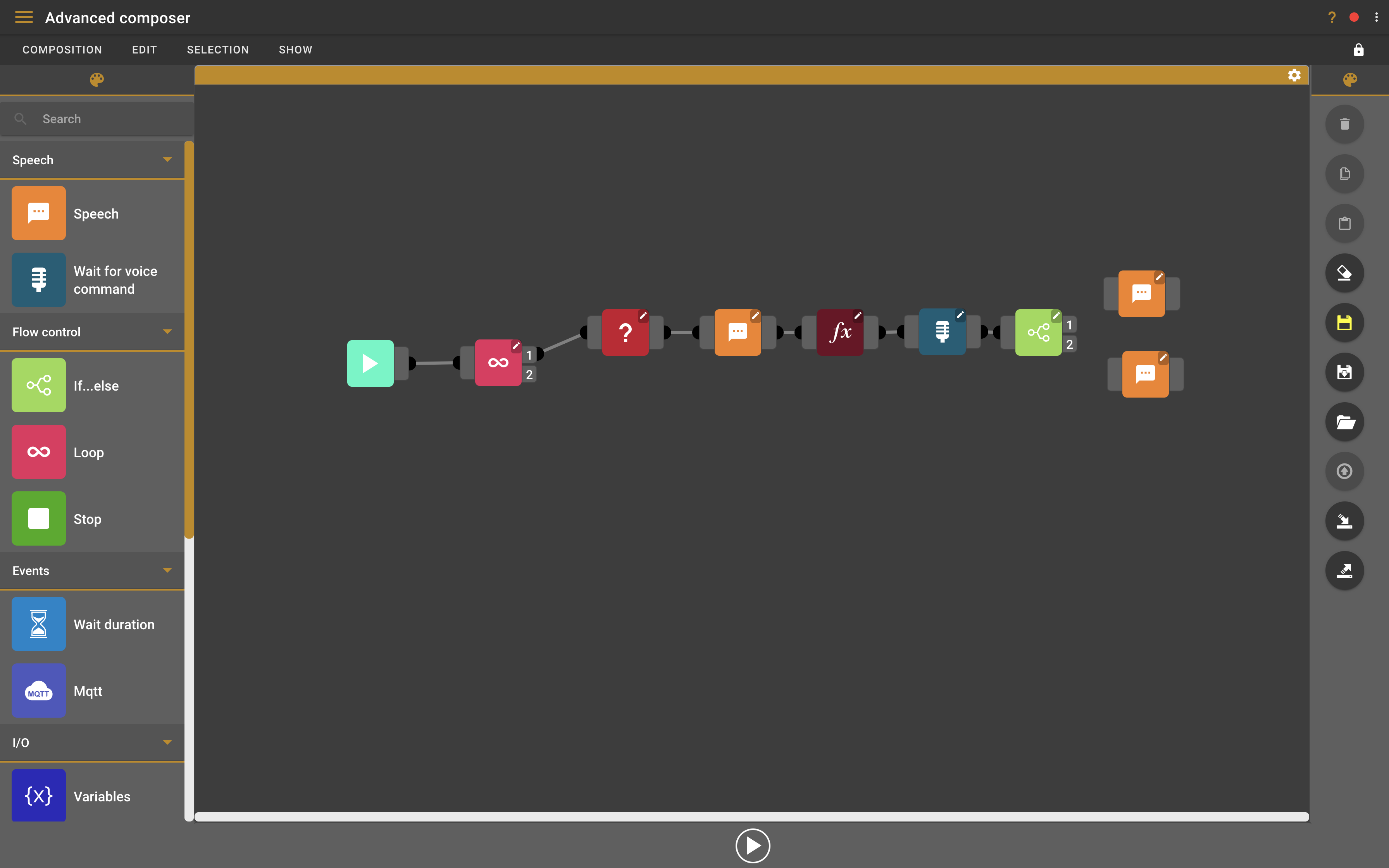8. We will add the following message to the first speech block "{result} is correct". We tell the user that his answer was correct while also repeating the result one more time. You can add this message to the speech block like we learned earlier in step 16 and 17 of this tutorial.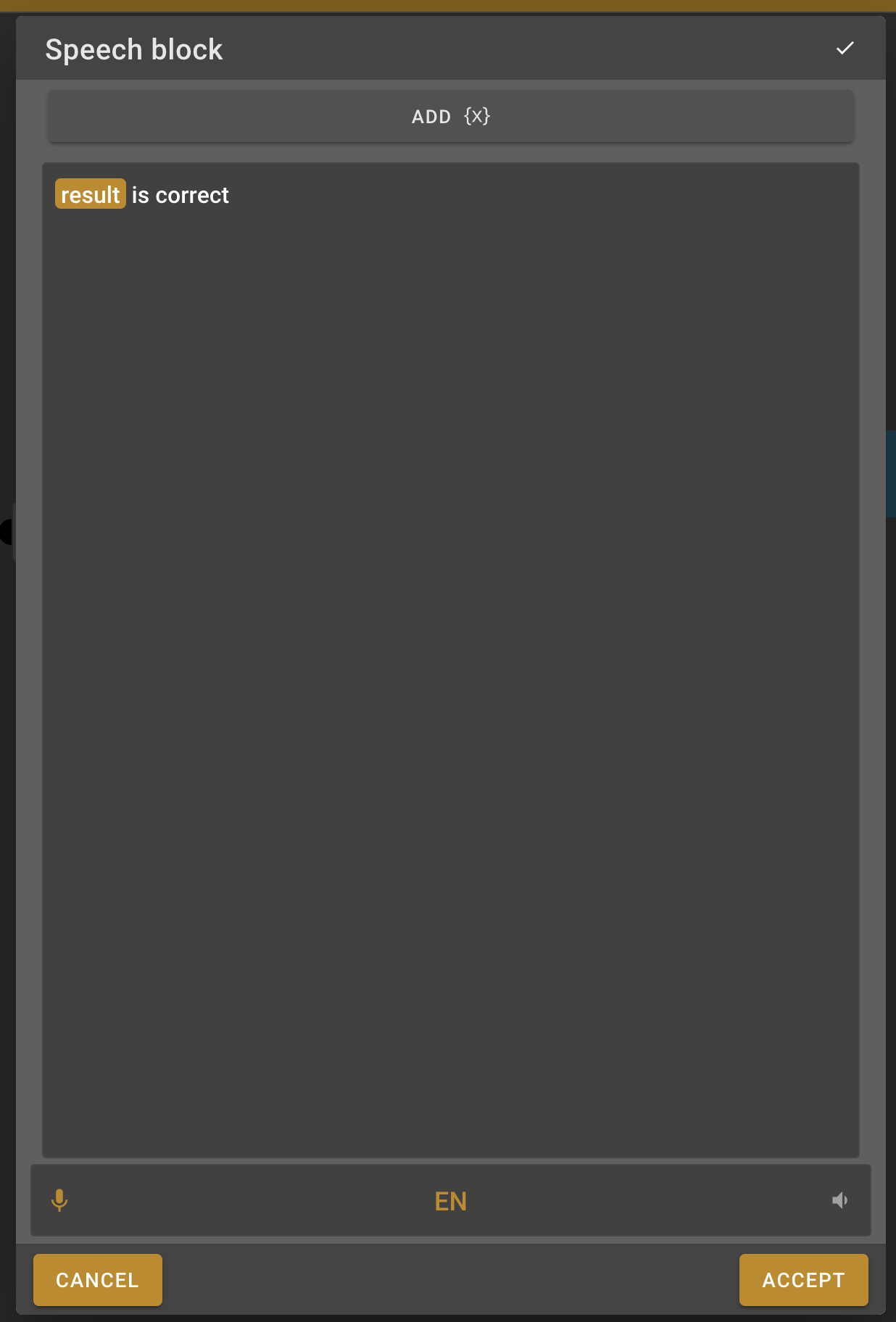9. We will do the same for the second node but here the message will let the user know that the result was incorrect. For example, you can write the following message in the speech block "Incorrect, the correct result was {result}"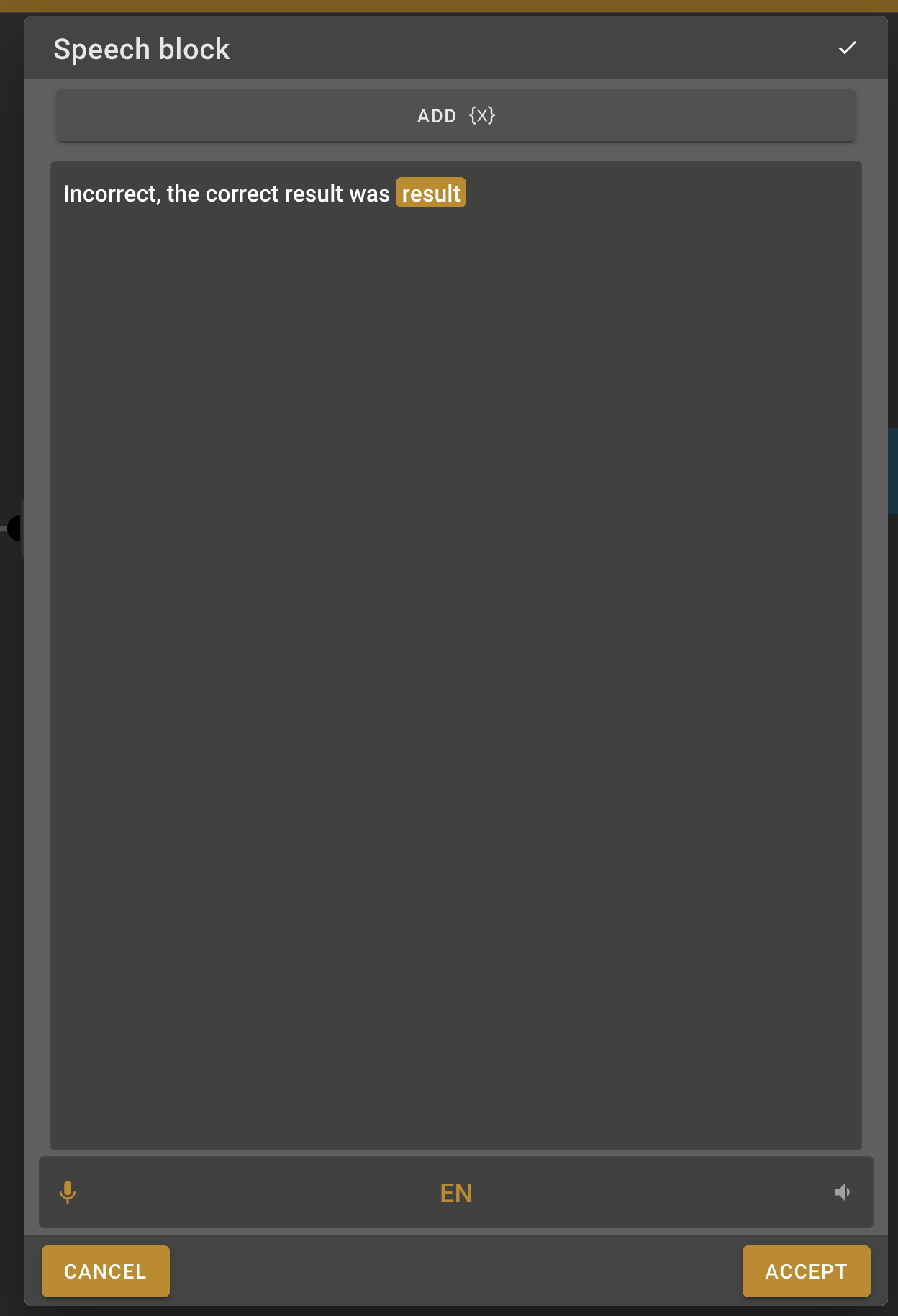### Stop block

1. To let the robot know that our composition is complete we need to add a Stop block. Find this block in the left menu and add it to the work field.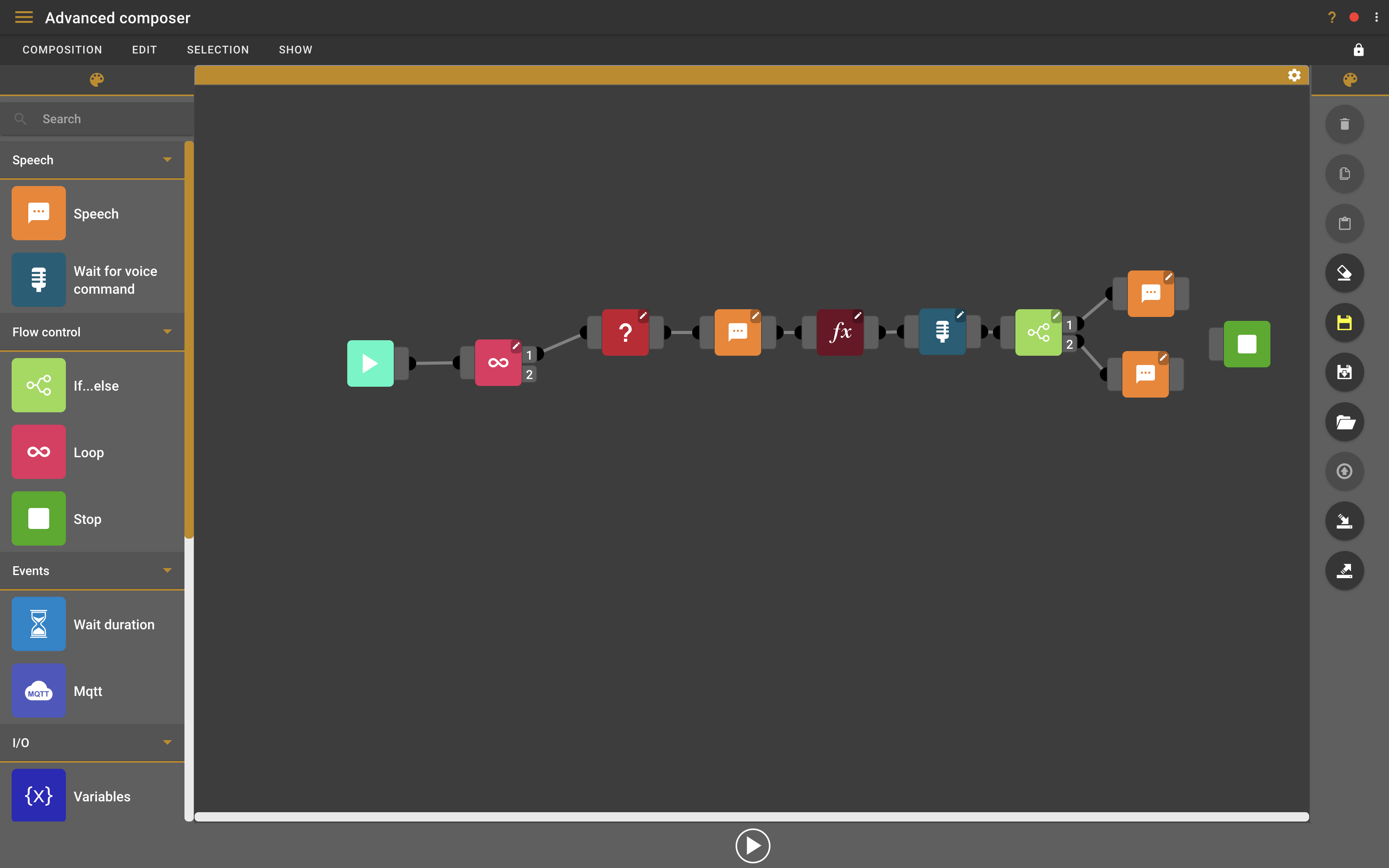2. Connect the stop block to both of the speech blocks. The first node of the loop node is now closed, when reaching this stop block the loop block will start the same flow over until it has done this 10 times like we told it to in the loop block settings. When we looped ten time it will follow the second node of the loop block.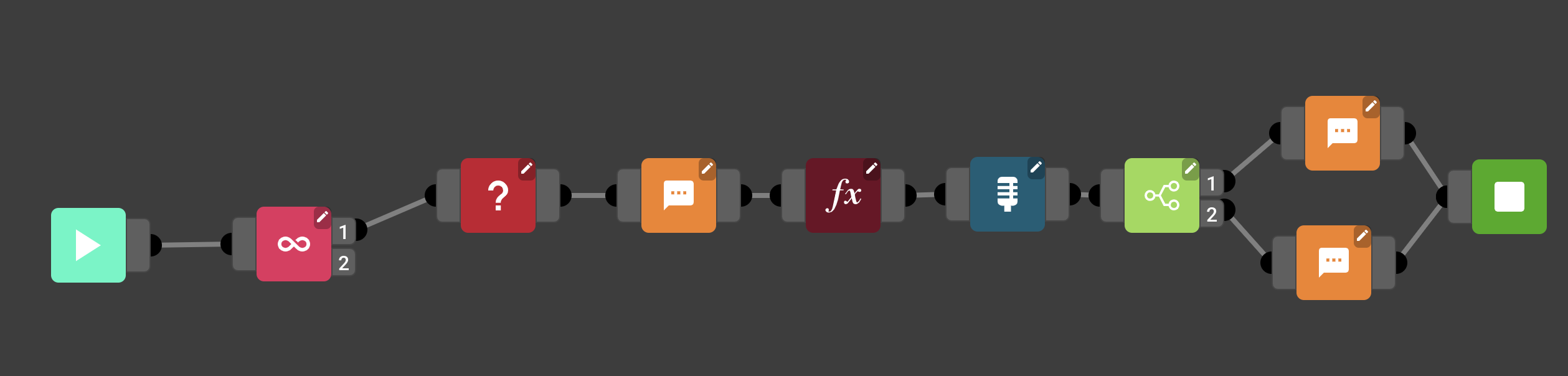3. The second loop node is still empty. We want to end the composition after we asked ten questions, to do this we only have to connect another stop block to the second node of the loop block.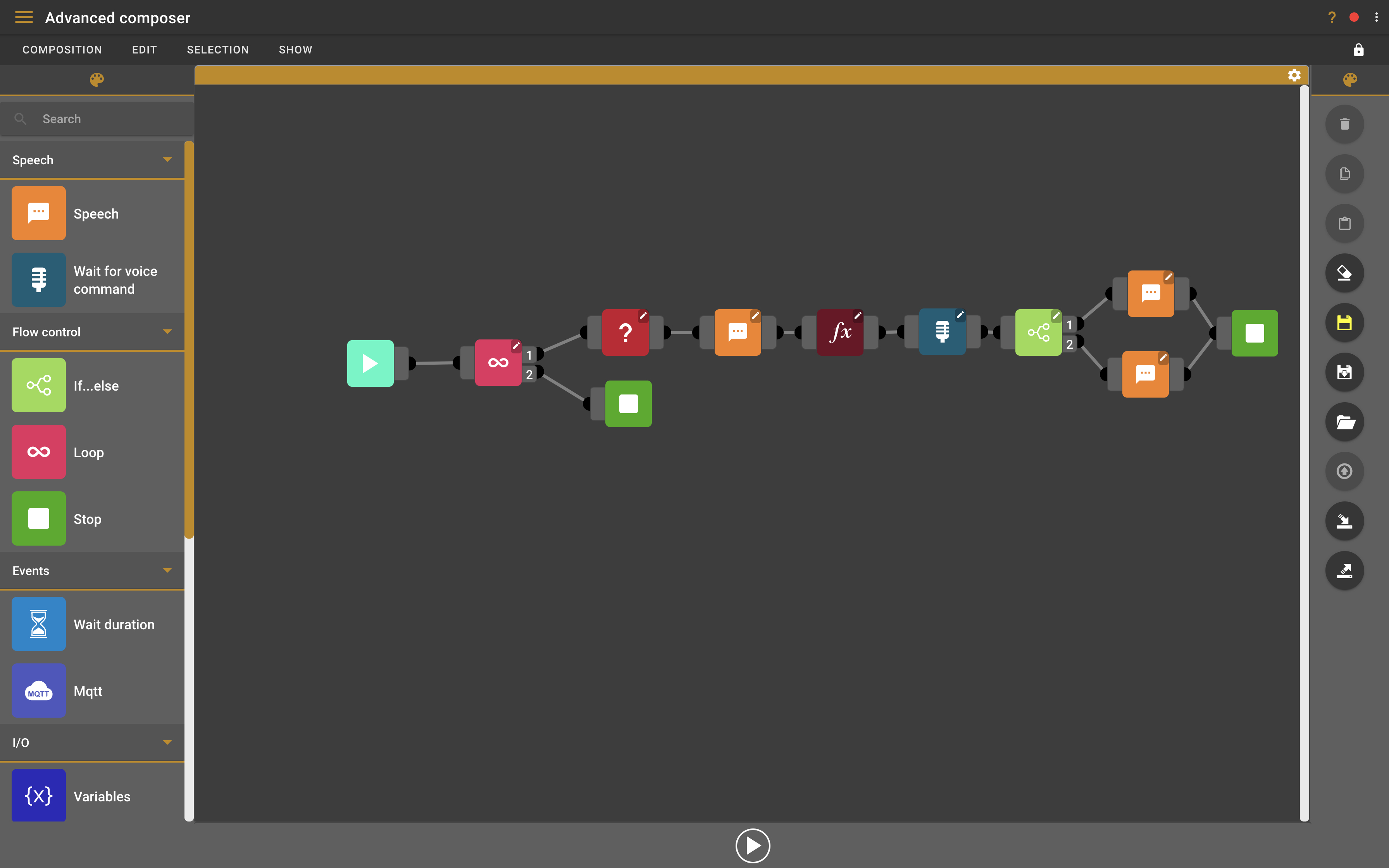### Play composition

1. To test the composition you can press the play button on the bottom of the composer.